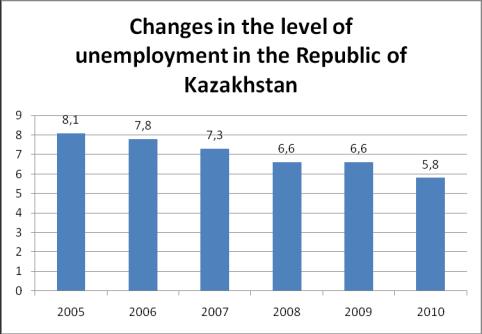Экономические науки/8. Математические методы в экономике

Serkebayeva L.T

A.Baitursynov Kostanai State University, Kazakhstan

Statistical methods for the analysis of employment and unemployment

The International Labor Statistics uses in his practice methods of the general theory of statistics. Аll processes in the field of labor relations are studied in dynamics and in the relationship.

Statistics developed a variety of methods to study relationships. Communication between processes and their characteristics are classified by the degree of closeness of the connection, direction and analytical expression.

To investigate the relationships using the method of comparing two parallel rows, analytical method groups, method of correlation tables, method of sample means, correlation analysis, regression analysis, index method and other.

To gather information International Labor Statistics uses a statistical survey. This method allows to obtain the necessary information on the developed program.

After receiving information is its control. International Labor Statistics uses the following types of control: syntax, arithmetic, logic.

After checking the quality of the resulting statistical information is a summary of her group.

Summary statistics is to obtain group and grand totals on program.  For example, by country.

The grouping is to divide the target population into homogeneous groups according to the studied traits. Indicators can be both quantitative and qualitative.

The method of comparing two parallel series is one of the simplest methods. This factors are arranged in ascending or descending order. Тhen observe the change in the resultant variable. Analysis arranged so that allows you to set the values ​​of the link and its destination.

The relationship between the factors and indicators can be tracked over time.

The method of sample means and the relative values ​​of held to disseminate the findings. Сonclusions reached by the sample data apply to the general population.

The method of average and relative quantities used to characterize the distribution of interval time series.

In international labor statistics are widely used index method. On the one hand, it allows to study the dynamics of Labor Statistics, оn the other hand, the index analysis allows to determine the quantitative effect of the factor on the result.

In the implementation of the classification of units together on several grounds International Labor Statistics uses cluster analysis. This method allows you to combine these units (countries or economic regions) in a relatively homogeneous groups (clusters). Еach unit in the cluster has a performance close to the group average.

A special place in the labor statistics is the balance method. It gives the opportunity to get milestones in between one-time survey. Balance method allows you to associate the presence of the working population with its use and to determine the excess or shortage of labor in the economy.

An example of the use of statistical methods in the analysis of unemployment.

Here are the following statistical calculations:

Ø      absolute increase;

Ø      growth rate;

Ø      rate of increase.

Calculated by the chain method.

Table 1.Figure 1.

Literature:

1.     Stat.kz – сайт Агентства Статистики Республики Казахстан

2.     Елисеева И.И. Статистика: УчебникМ: Проспект, 2005. – 443 с.

3.     Курышева С.В., Кашина О.Н. Статистическое изучение занятости и безработицы: Текст лекций – Издательство Санкт-Петербургского государственного университета экономики и финансов, 1997. – 85 с.